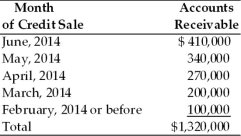/
/
/
21) If the cash discount period increased, a firm's investment
Not my Question
Flag Content

# Question : 21) If the cash discount period increased, a firm's investment : 1415279

21) If the cash discount period is increased, a firm's investment in accounts receivable is expected to ________.

A) increase because new customers attracted by the new policy will result in new accounts

receivable

B) decrease because new customers will doubt the quality of product due to increase in discount

C) increase because existing discount takers will pay more to get more discount

D) decrease because of existing discount takers will now pay earlier to avail the cash discount

22) Which of the following is true of changes in cash discount period?

A) If a firm increases its cash discount period, the sales are expected to decrease, the bad debts are expected to decrease, and the profit per unit is expected to increase.

B) If a firm decreases its cash discount period, the sales are expected to decrease, the bad debts are expected to decrease, and the profit per unit is expected to increase.

C) If a firm increases its cash discount period, the sales are expected to increase, the bad debts are expected to decrease, and the profit per unit is expected to decrease.

D) If a firm decreases its cash discount period, the sales are expected to decrease, the bad debts are expected to increase, and the profit per unit is expected to decrease.

23) Which of the following is true of changes in cash discount period?

A) If a firm decreases its cash discount period, the sales are expected to decrease, the bad debts are

expected to increase, and the profit per unit is expected to increase.

B) If a firm decreases its cash discount period, the sales are expected to increase, the bad debts are

expected to increase, and the profit per unit is expected to decrease.

C) If a firm increases its cash discount period, the sales are expected to decrease, the bad debts are expected to decrease, and the profit per unit is expected to increase.

D) If a firm increases its cash discount period, the sales are expected to increase, the bad debts are expected to decrease, and the profit per unit is expected to increase.

24) If a firm's credit period is increased, the sales volume can be expected to ________, the investment in accounts receivable can be expected to ________, and the bad debt expenses can be expected to ________.

A) increase; decrease; decrease

B) increase; increase; decrease

C) increase; increase; increase

D) decrease; decrease; decrease

25) If a firm's credit period is decreased, the sales volume can be expected to ________, the investment in accounts receivable can be expected to ________, and the bad debt expenses can be expected to ________.

A) increase; decrease; decrease

B) increase; increase; decrease

C) increase; increase; increase

D) decrease; decrease; decrease

Table 14.6

A breakdown of Teffan, Inc.'s outstanding accounts receivable dated June 30, 2014 on the basis of the month in which the credit sale was initially made follows. The firm extends 30-day credit terms.26) Accounts receivable due over 90 days total ________. (See Table 14.6)

A) \$200,000

B) \$470,000

C) \$300,000

D) \$100,000

27) An evaluation of the firm's collection efforts based on the aging schedule would suggest ________. (See Table 14.6)

A) poor credit management

B) satisfactory credit management

C) superior credit management

D) overzealous collection efforts

28) An increase in collection efforts by a firm will result in ________ in sales volume, ________ in the investment in accounts receivable, ________ in bad debt expenses, and ________ in collection expenditures.

A) an increase; a decrease; an increase; a decrease

B) an increase; a decrease; a decrease; an increase

C) an increase; a decrease; an increase; an increase

D) a decrease; a decrease; a decrease; an increase

29) A decrease in collection efforts by a firm will result in ________ in sales volume, ________ in the investment in accounts receivable, ________ in bad debt expenses, and ________ in collection expenditures.

A) an increase; an increase; an increase; a decrease

B) an increase; a decrease; an increase; an increase

C) an increase; a decrease; an increase; a decrease

D) a decrease; a decrease; a decrease; an increase

30) An increase in accounts receivable turnover for a firm due to an increase in collection efforts will ________.

A) decrease the firm's marginal investments in accounts receivable

B) increase the firm's marginal investments in accounts receivable

C) decrease the firm's collection expense

D) increase the firm's bad debt expense

## Solution 5 (1 Ratings )

Solved
Finance 1 Year Ago 53 Views
This Question has Been Answered!